Related Articles
Multi-Character Literal in C/C++
• Last Updated : 08 Mar, 2021

Character literals for C and C++ are char, string, and their Unicode and Raw type. Also, there is a multi-character literal that contains more than one c-char. A single c-char literal has type char and a multi-character literal is conditionally-supported, has type int, and has an implementation-defined value

Example:

```'a' is a character literal.
"abcd" is a string literal.
'abcd' is a multicharacter literal.```

Most C/C++ compilers support multi-character literals. Below is the example to demonstrate the concept of Multi-character Literal.

Example 1:

## C

 `// C program to demonstrate ``// Multicharacter literal``#pragma GCC diagnostic ignored "-Wmultichar"`` ` `// This disables the ``// multi-character warning``#include `` ` `// Driver code``int` `main()``{``  ``printf``(``"%d"``, ``'abcd'``);``  ``return` `0;``}`
Output

`1633837924`

Example 2:

## C++

 `// C++ program to demonstrate``// multi-character literal``#include `` ` `// Due to a bug we can't disable``// multi-character warning in C++``// using #pragma GCC diagnostic``// ignored "-Wmultichar"``using` `namespace` `std;`` ` `// Driver code``int` `main()``{``    ``cout << ``'abcd'` `<< endl;``    ``return` `0;``}`

Output: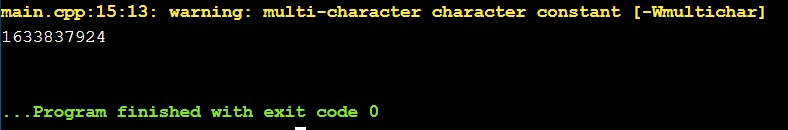This compiles and runs fine and the multi-character literal stores as an integer value (from where the number comes you will find below). As pedantic compiler flag generally passed it gives a warning on all multi-character literals. This warning helps to point out if we mistakenly use instead of .  The warning is:

`warning: multi-character character constant [-Wmultichar]`

You can disable the warning using the #pragma GCC diagnostic ignored “-Wmultichar” directly from the source code.

Below are some important information about Multi-character Literals:

1. Multi-character Literals are different from the string: Multi character literals are not the same as string or character array, they are totally different. Multi character literals are integer type’s not character types.

## C++

 `#include ``#include ``using` `namespace` `std;`` ` `int` `main()``{``    ``auto` `a = 10;``    ``auto` `b = ``'a'``;``    ``auto` `c = ``'abcd'``;``    ``auto` `d = ``"abcd"``;`` ` `    ``// 10 is of type i or int``    ``cout << ``"type of 10 is "``      ``<< ``typeid``(a).name() << endl;`` ` `    ``// 'a' is of type c or char``    ``cout << ``"type of \'a\' is "``      ``<< ``typeid``(b).name() << endl;`` ` `    ``// Multicharacter literals``    ``// 'abcd' is of type i or int``    ``cout << ``"type of \'abcd\' is "``      ``<< ``typeid``(c).name() << endl;`` ` `    ``// "abcd" is of type string``    ``cout << ``"type of \"abcd\" is "``      ``<< ``typeid``(d).name() << endl;`` ` `    ``return` `0;``}`

Output: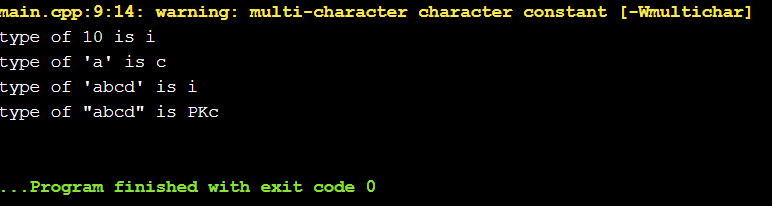Though typeid() should not be used to tell the type as it’s sometimes guaranteed by the standard to give you the wrong answer. But here typeid() is sufficient to point out that Multi-character stores as an integer type and different from char and string.

2. Multi-character literals are implementation-defined and not a bug:

An aspect of C++’s semantics that is defined for each implementation rather than specified in the standard for every implementation. An example is the size of an int (which must be at least 16 bits but can be longer). Avoid implementation-defined behavior whenever possible.

Any code that relies on implementation-defined behavior is only guaranteed to work under a specific platform and/or compiler.
Example:

• sizeof(int); It may be 4 bytes or 8 bytes depend on the compiler.
• int *p = malloc(0 * sizeof *o); It may result in p either being NULL or a unique pointer (as specified in 7.20.3 of the C99 Standard).

C++ inherited the multi-character literals from C and C inherited it from the B programming language. Most compilers (except MSVC) implement multi-character literals as specified in B

It is not that the creators of C or C++ didn’t know about this, they just leave it in hands of compilers to deal with it.

3. Multi-character literals stores as int rather than char (C standard): Now the question is from where the integer value is coming. On compilers where int is 4 bytes, Multi-characters stores as 4 bytes as it depends on the compiler.  For 4 bytes Multi-character literal each char initialize successive bytes of the resulting integer(big-endian, zero-padded, right-adjusted order). For Example, The value converted in 4 bytes int for ASCII char as,

Here, integers have 4 bytes of storage:

Now the ASCII of the first character from the left gets stored at last. Basically, “Big-endian” byte ordering:

Then for the next character, the integer value shifted 1 byte left:

And so on,

Now, these 4 bytes represent a single integer number and calculated as:

`'abcd' = (('a'*256 + 'b')*256 + `c`)*256 + 'd' = 1633837924 = 0x61626364 = '0xa0xb0xc0xd'`

## C++

 `#include ``using` `namespace` `std;`` ` `// Driver code``int` `main()``{``    ``cout << ``"\'abcd\' = "` `<< 'abcd' << ``" = "` `<< hex``         ``<< ``'abcd'` `<< endl;`` ` `    ``cout << ``"\'a' = "` `<< dec << (``int``)``'a'` `<< ``" = "` `<< hex``         ``<< (``int``)``'a'``;`` ` `    ``return` `0;``}`

Output: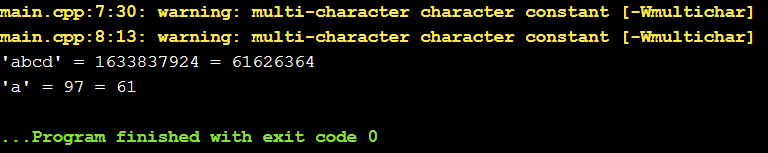If there are more than 4 characters in the Multi-character literal then only the last 4 characters stored and therefore, ‘abcdefgh’ == ‘efgh’, although the compiler will issue a warning on the literal that overflows.

## C++

 `#include ``using` `namespace` `std;`` ` `// Driver code``int` `main()``{``    ``cout << ``"\'abcd\' = "` `<< 'abcd' << endl;``    ``cout << ``"\'efgh\' = "` `<< 'efgh' << endl;``    ``cout << ``"\'abcdefgh\' = "` `<< 'abcdefgh' << endl;``    ``return` `0;``}`

Output: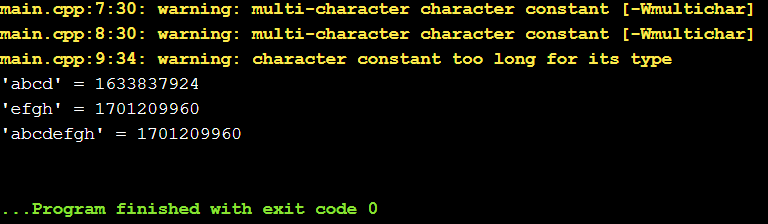And like above if we try to store Multi-character literal as char then only the last character gets stored.

## C++

 `#include ``using` `namespace` `std;`` ` `// Driver code``int` `main()``{``    ``char` `c = ``'abcd'``;`` ` `    ``// stores as, c = ' d '``    ``cout << c << endl;`` ` `    ``return` `0;``}`

Output: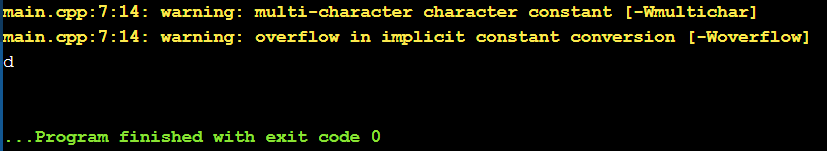Here we can see that the Multi-character literal which is a 4-byte integer is converting to 1-byte char and only stored the last character.

Good thing about multi-character literals: As multi-character literals are store as int, it can be used for comparison and in switch-case where strings are not used normally.

## C++

 `#include ``using` `namespace` `std;`` ` `// Driver code``int` `main()``{``    ``int` `s = ``'abcd'``;`` ` `    ``switch` `(s) {``    ``case` `'abcd'``:``        ``cout << (``'abcd'` `== ``'abcd'``);`` ` `        ``// s = 1633837924 and 'abcd'``        ``// = 1633837924 so, value of``        ``// s is equal to 'abcd'``    ``}`` ` `    ``return` `0;``}`

Output: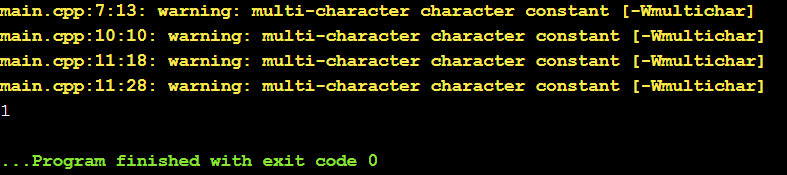Problems with multi-character literals:

• In C++, multi-character literals are conditionally-supported. Thus, your code may fail to compile.
• They are supported, but they have implementation-defined value. If the code runs on your machine fine that does not guarantee it will run on other machines.
• Also, it is possible that the implementation chooses to assign all multi-character literals the value 0, breaking your code.
Want to learn from the best curated videos and practice problems, check out the C++ Foundation Course for Basic to Advanced C++ and C++ STL Course for foundation plus STL.  To complete your preparation from learning a language to DS Algo and many more,  please refer Complete Interview Preparation Course.
My Personal Notes arrow_drop_up